# Comparing samples post-matching – some helper functions after FUZZY (SPSS)

I’ve been conducting quite a few case-control or propensity score matching studies lately. So I wrote some helper functions for use after the SPSS `FUZZY` command. These create the case-control dataset, plus calculate some of the standardized bias metrics for matching on continuous outcomes.

The use case here is if you have a sub-set of treated individuals, and you want to draw a comparison sample matched on certain characteristics (which can include just one propensity score and/or multiple covariates). Here is the macro to follow along, and I will provide a quick walkthrough of how it works. (There is documentation in the header for what the parameters are and what the function returns.)

So first I am going to import my macro using `INSERT`:

``````*Inserting the macro.
INSERT FILE = "C:\Users\andrew.wheeler\Dropbox\Documents\BLOG\Matching_StandBias\PropBalance_Macro.sps".``````

Now just for illustration I am going to make a fake dataset to illustrate the utility of matching. Here I have a universe of 2,000 people. There is a subset of treated individuals (165), but they are only selected if they are under 28 years old and male.

``````*Create a fake dataset.
SET SEED 10.
INPUT PROGRAM.
LOOP Id = 1 TO 2000.
END CASE.
END LOOP.
END FILE.
END INPUT PROGRAM.
DATASET NAME OrigData.
COMPUTE Male = RV.BERNOULLI(0.7).
COMPUTE YearsOld = RV.UNIFORM(18,40).
FORMATS Male (F1.0) YearsOld (F2.0).
DO IF Male = 1 AND YearsOld <= 28.
COMPUTE Treated = RV.BERNOULLI(0.3).
ELSE.
COMPUTE Treated = 0.
END IF.
COMPUTE #OutLogit = 0.7 + 0.5*Male - 0.05*YearsOld - 0.7*Treated.
COMPUTE #OutProb = 1/(1 + EXP(-#OutLogit)).
COMPUTE Outcome = RV.BERNOULLI(#OutProb).
FREQ Treated Outcome.``````

So what happens when we make comparisons among the entire sample, which includes females and older people?

``````*Compare means with the original full sample.
T-TEST GROUPS=Treated(0 1) /VARIABLES=Outcome.``````

We get basically no difference, our treated mean is 0.40 and the untreated mean is 0.39. But instead of comparing the 165 to the entire sample, we draw more reasonable control cases. Here we do an exact match on `Male`, and then we do a fuzzy match on `YearsOld` to within 3 years.

``````*Draw the comparison sample based on Male (exact) and YearsOld (Fuzzy).
FUZZY BY=Male YearsOld SUPPLIERID=Id NEWDEMANDERIDVARS=Match1 GROUP=Treated
EXACTPRIORITY=FALSE FUZZ=0 3 MATCHGROUPVAR=MGroup DRAWPOOLSIZE=CheckSize
/OPTIONS SAMPLEWITHREPLACEMENT=FALSE MINIMIZEMEMORY=TRUE SHUFFLE=TRUE SEED=10.``````

Now what the `FUZZY` command does in SPSS is creates a new variable, named `Match1` here, that places the matched Id in the same row as the original treated sample. You cannot easily make the updated comparisons that you want though in this data format. So after writing the code to do this about 7 times, I decided to make it into a simple macro. Here is an example of calling my macro, `!MatchedSample`.

``````*Now run my macro to make the matched sample.
!MatchedSample Dataset=OrigData Id=Id Case=Treated MatchGroup=MGroup Controls=[Match1]
MatchVars=[YearsOld] OthVars=Outcome Male.``````

This then spits out two new datasets, as well as appends a new variable to the original dataset named `MatchedSample` to show what cases have been matched. Then it is simple to see the difference in our means among our matched sample.

``````*Now the t-test with the matched sample subset.
DATASET ACTIVATE MatchedSamples.
T-TEST GROUPS=Treated(0 1) /VARIABLES=Outcome.``````

Which shows the same mean for treated, 0.40 (since all the treated were matched), but the comparison group now has a mean of 0.51, so here the treatment reduced the outcome.

The macro also provides an additional dataset named `AggStats` that estimates the standardized bias in the original sample vs. the standardized bias in the matched sample. (Standardized bias is just Cohen’s D measure multiplied by 100.) This then also calculates the standardized bias reduction for each continuous covariate. Before I forget, a neat way to test for balance jointly (instead of one variable at a time) is to conduct an additional regression equation predicting treatment and then testing for all coefficients equal to zero.

In this fake example the propensity scores would not be needed, you could just estimate a typical logistic regression equation controlling for `YearsOld` and `Male`. But the utility of matching comes from when you don’t know the functional form of how those covariates affect the outcome. So if the outcome was a very non-linear function of age, you don’t have to worry about estimating that function, you can just match on age and still get a reasonable comparison of the mean difference for treated vs. not-treated.

# Translating between the dispersion term in a negative binomial regression and random variables in SPSS

NOTE!! – when I initially posted this I was incorrect, I thought SPSS listed the dispersion term in the form of `Var(x) = mean + mean*dispersion`. But I was wrong, and it is `Var(x) = 1 + mean*dispersion` (the same as Stata’s, what Cameron and Trivedi call the NB2 model, as cited in the Long and Freese Stata book for categorical variables.) The simulation in the original post worked out because my example I used the mean as 1, here I update it to have a mean of 2 to show the calculations are correct. (Also note that this parametrization is equivalent to `Var(x) = mean*(1 + mean*dispersion)`, see Stata’s help for nbreg.)

When estimating a negative binomial regression equation in SPSS, it returns the dispersion parameter in the form of:

``Var(x) = 1 + mean*dispersion``

When generating random variables from the negative binomial distribution, SPSS does not take the parameters like this, but the more usual N trials with P successes. Stealing a bit from the R documentation for `dnbinom`, I was able to translate between the two with just a tedious set of algebra. So with our original distribution being:

``````Mean = mu
Variance = 1 + mu*a``````

R has an alternative representation closer to SPSS’s based on:

``````Mean = mu
Variance = mu + mu^2/x``````

Some tedious algebra will reveal that in this notation `x = mu^2/(1 - mu + a*mu)` (note to future self, using Solve in Wolfram Alpha could have saved some time, paper and ink). Also, R’s help for `dbinom` states that in the original N and P notation that `p = x/(x + mu)`. So here with `mu` and `a` (again `a` is the dispersion term as reported by GENLIN in SPSS) we can solve for `p`.

``````x = mu^2/(1 - mu + a*mu)
p = x/(x + mu)``````

And since `p` is solved, R lists the mean of the distribution in the N and P notation as:

``n*(1-p)/p = mu``

So with `p` solved we can figure out N as equal to:

``mu*p/(1-p) = n``

So to reiterate, if you have a mean of 2 and dispersion parameter of 4, the resultant N and P notation would be:

``````mu = 2
a = 4
x = mu^2/(1 - mu + a*mu) = 2^2/(1 - 2 + 4*2) = 4/7
p = x/(x + mu) = (4/7)/(4/7 + 2) = 2/9
n = mu*p/(1-p) = 2*(2/9)/(7/9) = 4/7``````

Here we can see that in the N and P notation the similar negative binomial model results in a fractional number of successes, which might be a surprising result for some that it is even a possibility. (There is likely an easier way to do this translation, but forgive me I am not a mathematician!)

Now we would be finished, but unfortunately SPSS’s negative binomial random functions only take integer values and do not take values of N less than 1 (R’s `dnbinom` does). So we have to do another translation of the N and P notation to the gamma distribution to be able to draw random numbers in SPSS. Another representation of the negative binomial model is a mixture of Poisson distributions, with the distribution of the mixtures being from a gamma distribution. Wikipedia lists a translation from the N and P notation to a gamma with shape = N and scale = P/(1-P).

So I wrapped these computations up in an SPSS macros that takes the mean and the dispersion parameter, calculates N and P under the hood, and then draws a random variable from the associated negative binomial distribution.

``````DEFINE !NegBinRV (mu = !TOKENS(1)
/disp = !TOKENS(1)
/out = !TOKENS(1) )
COMPUTE #x = !mu**2/(1 - !mu + !disp*!mu).
COMPUTE #p = #x / (#x + !mu).
COMPUTE #n = !mu*#p/(1 - #p).
COMPUTE #G = RV.GAMMA(#n,#p/(1 - #p)).
COMPUTE !Out = RV.POISSON(#G).
FORMATS !Out (F5.0).
!ENDDEFINE.``````

I am not sure if it is possible to use this gamma representation and native SPSS functions to calculate the corresponding CDF and PDF of the negative binomial distribution. But we can use R to do that. Here is an example of keeping the mean at 1 and varying the dispersion parameter between 0 and 5.

``````BEGIN PROGRAM R.
library(ggplot2)
x <- expand.grid(0:10,1:5)
names(x) <- c("Int","Disp")
mu <- 1
x\$PDF <- mapply(dnbinom, x=x\$Int, size=mu^2/(1 - mu + x\$Disp*mu), mu=mu)
t <- data.frame(cbind(0:10,rep(0,11),dpois(0:10,lambda=1)))
names(t) <- c("Int","Disp","PDF")
x <- rbind(t,x)
p <- ggplot(data = x, aes(x = Int, y = PDF, group = as.factor(Disp))) + geom_line()
p
#for the CDF
x\$CDF <- ave(x\$PDF, x\$Disp, FUN = cumsum)
END PROGRAM.``````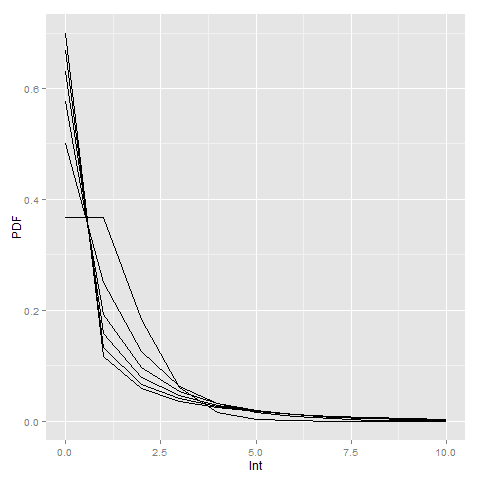Here you can see how the larger dispersion term can easily approximate the zero inflation typical in criminal justice data (see an applied example from my work). R will not take a dispersion parameter of zero in this notation (as the size would be divided by zero and not defined), so I just tacked on the Poisson distribution with a mean of zero.

Here is an example of generating random data from a negative binomial distribution with a mean of 2 and a dispersion parameter of 4. I then grab the PDF from R, and superimpose them both on a chart in SPSS (or perhaps I should call it a PMF, since it only has support on integer values). You can see the simulation with 10,000 observations is a near perfect fit (so a good sign I did not make any mistakes!)

``````*Simulation In SPSS.
INPUT PROGRAM.
LOOP Id = 1 TO 10000.
END CASE.
END LOOP.
END FILE.
END INPUT PROGRAM.
DATASET NAME RandNB.

!NegBinRV mu = 2 disp = 4 out = NB.

*Making seperate R dataset of PDF.
BEGIN PROGRAM R.
mu <- 2
disp <- 4
x <- 0:11
pdf <- dnbinom(x=x,size=mu^2/(1 - mu + disp*mu),mu=mu)
pdf[max(x)+1] <- 1 - sum(pdf[-(max(x)+1)])
MyDf <- data.frame(cbind(x,pdf))
END PROGRAM.
EXECUTE.
STATS GET R FILE=* /GET DATAFRAME=MyDf DATASET=PDF_NB.
DATASET ACTIVATE PDF_NB.
FORMATS x (F2.0).
VALUE LABELS x 11 '11 or More'.

*Now superimposing bar plot and PDF from separate datasets.
DATASET ACTIVATE RandNB.
RECODE NB (11 THRU HIGHEST = 11)(ELSE = COPY) INTO NB_Cat.
FORMATS NB_Cat (F2.0).
VALUE LABELS NB_Cat 11 '11 or More'.

GGRAPH
/GRAPHDATASET NAME="Data" DATASET='RandNB' VARIABLES=NB_Cat[LEVEL=ORDINAL] COUNT()[name="COUNT"]
/GRAPHDATASET NAME="PDF" DATASET='PDF_NB' VARIABLES=x pdf
/GRAPHSPEC SOURCE=INLINE.
BEGIN GPL
SOURCE: Data=userSource(id("Data"))
DATA: NB_Cat=col(source(Data), name("NB_Cat"), unit.category())
DATA: COUNT=col(source(Data), name("COUNT"))
SOURCE: PDF=userSource(id("PDF"))
DATA: x=col(source(PDF), name("x"), unit.category())
DATA: den=col(source(PDF), name("pdf"))
TRANS: den_per = eval(den*100)
GUIDE: axis(dim(1))
GUIDE: axis(dim(2))
SCALE: linear(dim(2), include(0))
ELEMENT: interval(position(summary.percent(NB_Cat*COUNT)), shape.interior(shape.square))
ELEMENT: point(position(x*den_per), color.interior(color.black), size(size."8"))
END GPL.``````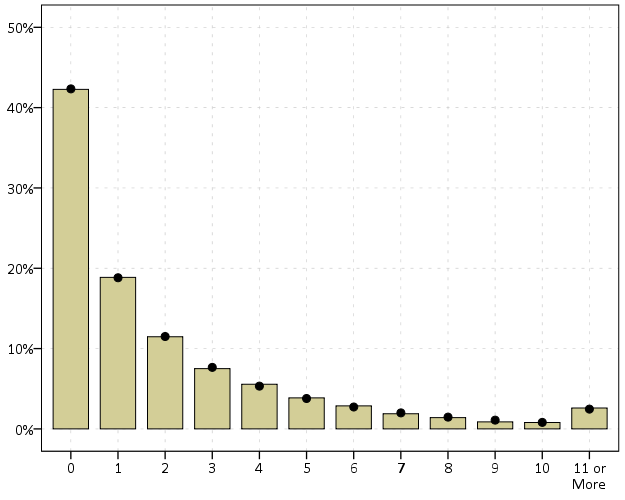# Treemaps in SPSS

Instead of an Xmas tree this year I will discuss a bit about treemaps. Treemaps are a visualization developed by Ben Shneiderman to identify how the current space on ones hard drive is being partitioned. Now they are a popular tool to visualize any hierarchical data that have quantitative size data associated with it. Some of my favorites are from Catherine Mulbrandon of the Visualizing Economics blog. Here is one visualizing the job market sector:There are quite a few problems with visualizing treemaps, mainly that evaluating areas are a much more difficult task than evaluating the position along an aligned axis. I find some of them visually appealing though, and well suited for their original goal: identifying large categories in unordered hierarchical data with very many categories. So I took some time to write up code to make them in SPSS. The layout algorithm I use (I believe) is the slice and dice, which does not look nice if there are many small categories, but basically a nice workaround is to create different levels in the hierarchy. (This took me about 4+ hours to do, and at this point I would just use a Python or R library to make them if I wanted a different layout algorithm.)

So here is the macro in an sps file (plus the other files used in this post), and it takes as parameters:

• `Data`: the name of the original dataset
• `Val` (optional): If your categorical data have a numeric variable indicating the size of the category. If not, it simply counts up the number of times a category is in the data file.
• `Vars`: the categorical variables that define the treemap. (This should work with as many categories as you want, tested currently with up to 4.)

So lets make some fake data, load in the macro, and then see what it spits out.

``````FILE HANDLE data /NAME = "C:\Users\andrew.wheeler\Dropbox\Documents\BLOG\TreeMaps_SPSS".
INSERT FILE = "data\TreeMap_MACRO.sps".
*Making some random data.
SET SEED 10.
INPUT PROGRAM.
LOOP #i = 1 TO 1000.
COMPUTE Cat1 = RV.UNIFORM(0,1).
COMPUTE Cat2 = RV.UNIFORM(0,1).
END CASE.
END LOOP.
END FILE.
END INPUT PROGRAM.
DATASET NAME Tree.
NUMERIC C1 C2.
DO REPEAT Prop1 = 0.6 0.9 0.97 1
/Prop2 = 0.4 0.7 0.9 1
/i = 1 TO 4.
IF (MISSING(C1) AND Cat1 <= Prop1) C1 = i.
IF (MISSING(C2) AND Cat2 <= Prop2) C2 = i.
END REPEAT.
COMPUTE C3 = RV.BERNOULLI(0.8).
MATCH FILES FILE = * /DROP Cat1 Cat2.
FORMATS C1 C2 C3 (F1.0).
EXECUTE.
*Making the rectangles.
!TreeMap Data = Tree Vars = C1 C2 C3.``````

You have returned a second dataset named `Tree_C3` that contains the corners of the boxes for each level of the hierarchy in a set of variables `BL_x, BL_y, TR_x, TR_y` (meant to be bottom left x, top right y etc.) Using the `link.hull` parameter for a polygon element in inline GPL (as I showed for spineplots) we can now create the boxes.

``````*Now plotting the rectangles.
MATCH FILES FILE = *
/FIRST = Flag
/BY C1 C2.
DO IF Flag = 0.
DO REPEAT x = BL_x2 BL_y2 TR_x2 TR_y2.
COMPUTE x = \$SYSMIS.
END REPEAT.
END IF.
*Prevents repeated drawing of the same polygon at a higher level.
EXECUTE.
GGRAPH
/GRAPHDATASET NAME="graphdataset" VARIABLES=BL_x3 BL_y3 TR_x3 TR_y3 BL_x2 BL_y2 TR_x2 TR_y2 C1 C2 C3
MISSING=VARIABLEWISE
/GRAPHSPEC SOURCE=INLINE TEMPLATE = "data\Labels_Poly.sgt".
BEGIN GPL
PAGE: begin(scale(800px,600px))
SOURCE: s=userSource(id("graphdataset"))
DATA: BL_x3=col(source(s), name("BL_x3"))
DATA: BL_y3=col(source(s), name("BL_y3"))
DATA: TR_x3=col(source(s), name("TR_x3"))
DATA: TR_y3=col(source(s), name("TR_y3"))
DATA: BL_x2=col(source(s), name("BL_x2"))
DATA: BL_y2=col(source(s), name("BL_y2"))
DATA: TR_x2=col(source(s), name("TR_x2"))
DATA: TR_y2=col(source(s), name("TR_y2"))
DATA: C1=col(source(s), name("C1"), unit.category())
DATA: C2=col(source(s), name("C2"), unit.category())
DATA: C3=col(source(s), name("C3"), unit.category())
TRANS: casenum = index()
SCALE: cat(aesthetic(aesthetic.texture.pattern), map(("0",texture.pattern.mesh),("1",texture.pattern.solid)))
GUIDE: legend(aesthetic(aesthetic.color.interior), label("Cat 1"))
GUIDE: legend(aesthetic(aesthetic.texture.pattern), label("Cat 3"))
GUIDE: axis(dim(1), null())
GUIDE: axis(dim(2), null())
ELEMENT: polygon(position(link.hull((BL_x3 + TR_x3)*(BL_y3 + TR_y3))), split(C2), color.interior(C1),
texture.pattern(C3))
ELEMENT: polygon(position(link.hull((BL_x2 + TR_x2)*(BL_y2 + TR_y2))), transparency.exterior(transparency."1"),
transparency.interior(transparency."1"), label(C2), split(casenum))
ELEMENT: edge(position(link.hull((BL_x2 + TR_x2)*(BL_y2 + TR_y2))), size(size."3"), split(casenum))
PAGE: end()
END GPL.``````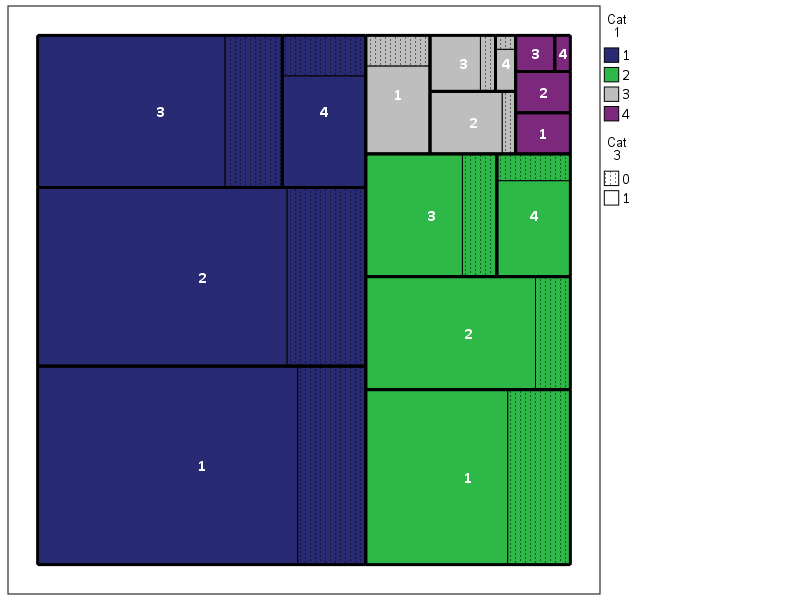So here is a quick rundown of the complicated GPL code. Here I mapped colors to the first `C1` category, and then made `C3` a different texture pattern. To get all of the squares to draw I use the `split` modifier on the `C2` category, which has no direct aesthetics mapped, and then placed the C2 label in the center. I made the labels white with an additional chart template to my default, and made the outline for the C2 bars more distinct by plotting them on top as an edge element and making them thicker. If I wanted to publish this, I would probably just export the vector chart and add in the labels in nice spots (SPSS I don’t believe you can style the labels separately, maybe you can with some chart template magic that I am unaware of). So here if I could in SPSS I would make the labels for category 1 in the top left of its respective color, but that is not possible.

If we change the categories to not be so uneven, you can see how my slice-and-dice layout algorithm is not so nice. Here it is with the proportions being about equal for all categories.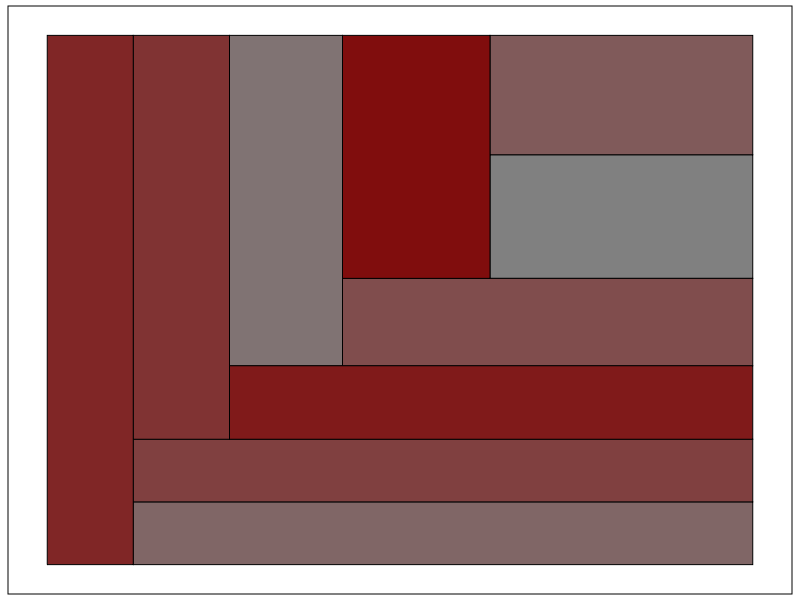Fortunately most categorical data are not like this, and have uneven distributions (also with even data and more hierarchies it tends to look nicer). For an actual example, I grabbed the NIBRS 2012 incident data from ICPSR, which is incident level crime reports from participating police jurisdictions over the country (NIBRS stands for National Incident Based Reporting System). It is pretty big, over 5 million records, and with the over 350 variables the fixed text file is over 6 gigabytes, but the compressed zsav format is only slightly larger than the original gzipped file from ICPSR, (0.35 gigabytes vs 0.29 gigabytes in the fixed width ascii gzipped). So here I grab the NIBRS data I prepared, and create the hierarchy as follows:

• Level 1: I aggregate the UCR crimes into Part 1 Violent, Part 1 Non-Violent, and Other
• Level 2: Individual UCR categories
• Level 3: Location Type broken down into outdoor, indoor, home, and other/missing

And here is the code and the plot:

``````*Now NIBRS data.
DATASET CLOSE ALL.
GET FILE = "data\NIBRS_2012.zsav".
DATASET NAME NIBRS_2012.

RECODE V20061 (91 THRU 132 = 1)(200 THRU 240 = 2)(ELSE = 3) INTO UCR_Cat.
VALUE LABELS UCR_Cat 1 'Violent' 2 'Property' 3 'Other'.
RECODE V20111 (10,13,16,18,50,51 = 1)(20 = 3)(25, LO THRU 0 = 4)(ELSE = 2) INTO Loc_Cat.
VALUE LABELS Loc_Cat 1 'Outdoor' 2 'Indoor' 3 'Home' 4 'Other'.

!TreeMap Data = NIBRS_2012 Vars = UCR_Cat V20061 Loc_Cat.
DATASET ACTIVATE Tree_Loc_Cat.

MATCH FILES FILE = *
/FIRST = Flag_UCRType
/BY UCR_Cat V20061.
DO IF Flag_UCRType = 0.
DO REPEAT x = BL_x2 BL_y2 TR_x2 TR_y2.
COMPUTE x = \$SYSMIS.
END REPEAT.
END IF.

*Calculating width, if under certain value not placing label.
MATCH FILES FILE = * /DROP UCR_Lab.
STRING UCR_Lab (A20).
IF (TR_x2 - BL_x2) >= 0.12 UCR_Lab = VALUELABEL(V20061).
EXECUTE.

GGRAPH
/GRAPHDATASET NAME="graphdataset" VARIABLES=BL_x3 BL_y3 TR_x3 TR_y3 UCR_Cat UCR_Lab Loc_Cat
BL_x2 BL_y2 TR_x2 TR_y2 MISSING=VARIABLEWISE
/GRAPHSPEC SOURCE=INLINE TEMPLATE = "data\Labels_Poly.sgt".
BEGIN GPL
PAGE: begin(scale(800px,600px))
SOURCE: s=userSource(id("graphdataset"))
DATA: BL_x3=col(source(s), name("BL_x3"))
DATA: BL_y3=col(source(s), name("BL_y3"))
DATA: TR_x3=col(source(s), name("TR_x3"))
DATA: TR_y3=col(source(s), name("TR_y3"))
DATA: BL_x2=col(source(s), name("BL_x2"))
DATA: BL_y2=col(source(s), name("BL_y2"))
DATA: TR_x2=col(source(s), name("TR_x2"))
DATA: TR_y2=col(source(s), name("TR_y2"))
DATA: UCR_Cat=col(source(s), name("UCR_Cat"), unit.category())
DATA: UCR_Lab=col(source(s), name("UCR_Lab"), unit.category())
DATA: Loc_Cat=col(source(s), name("Loc_Cat"))
TRANS: casenum = index()
SCALE: linear(aesthetic(aesthetic.color.saturation.interior), aestheticMaximum(color.saturation."1"),
aestheticMinimum(color.saturation."0.4"))
GUIDE: legend(aesthetic(aesthetic.color.interior), null())
GUIDE: legend(aesthetic(aesthetic.color.saturation.interior), null())
GUIDE: axis(dim(1), null())
GUIDE: axis(dim(2), null())
ELEMENT: polygon(position(link.hull((BL_x3 + TR_x3)*(BL_y3 + TR_y3))),
color.interior(UCR_Cat), split(casenum), transparency.exterior(transparency."1"),
color.saturation.interior(Loc_Cat))
ELEMENT: polygon(position(link.hull((BL_x2 + TR_x2)*(BL_y2 + TR_y2))),
transparency.exterior(transparency."1")), transparency.interior(transparency."1"),
label(UCR_Lab), split(casenum))
ELEMENT: edge(position(link.hull((BL_x2 + TR_x2)*(BL_y2 + TR_y2))), size(size."3"), split(casenum))
PAGE: end()
END GPL.``````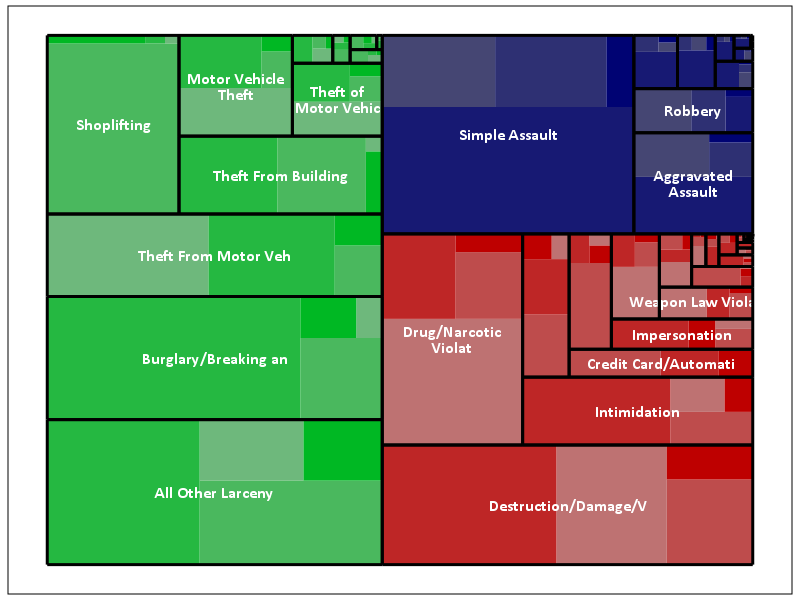The saturation for locations types goes from lightest to darkest: Outdoor, Indoor, Home, Other. Instead of randomly allocating the saturation to distinguish between the location types furthest down the hierarchy, I can map the saturation to another category. Here I map it to whether someone was arrested for the proportion of offenses.

``````*Adding in proportion of arrests.
DATASET ACTIVATE NIBRS_2012.
COMPUTE Arrest = (RECSARR > 0).
DATASET DECLARE ArrestProp.
AGGREGATE OUTFILE='ArrestProp'
/BREAK UCR_Cat V20061 Loc_Cat
/ArrestProp = MEAN(Arrest).
DATASET ACTIVATE Tree_Loc_Cat.
MATCH FILES FILE = *
/TABLE = 'ArrestProp'
/BY UCR_Cat V20061 Loc_Cat.
DATASET CLOSE ArrestProp.

*Now mapping arrest proportion to saturation.
GGRAPH
/GRAPHDATASET NAME="graphdataset" VARIABLES=BL_x3 BL_y3 TR_x3 TR_y3 UCR_Cat UCR_Lab Loc_Cat ArrestProp
BL_x2 BL_y2 TR_x2 TR_y2 MISSING=VARIABLEWISE
/GRAPHSPEC SOURCE=INLINE TEMPLATE = "data\Labels_Poly.sgt".
BEGIN GPL
PAGE: begin(scale(800px,600px))
SOURCE: s=userSource(id("graphdataset"))
DATA: BL_x3=col(source(s), name("BL_x3"))
DATA: BL_y3=col(source(s), name("BL_y3"))
DATA: TR_x3=col(source(s), name("TR_x3"))
DATA: TR_y3=col(source(s), name("TR_y3"))
DATA: BL_x2=col(source(s), name("BL_x2"))
DATA: BL_y2=col(source(s), name("BL_y2"))
DATA: TR_x2=col(source(s), name("TR_x2"))
DATA: TR_y2=col(source(s), name("TR_y2"))
DATA: UCR_Cat=col(source(s), name("UCR_Cat"), unit.category())
DATA: UCR_Lab=col(source(s), name("UCR_Lab"), unit.category())
DATA: Loc_Cat=col(source(s), name("Loc_Cat"))
DATA: ArrestProp=col(source(s), name("ArrestProp"))
TRANS: casenum = index()
SCALE: linear(aesthetic(aesthetic.color.saturation.interior), aestheticMaximum(color.saturation."1"),
aestheticMinimum(color.saturation."0.4"))
GUIDE: legend(aesthetic(aesthetic.color.interior), null())
GUIDE: legend(aesthetic(aesthetic.color.saturation.interior), null())
GUIDE: axis(dim(1), null())
GUIDE: axis(dim(2), null())
ELEMENT: polygon(position(link.hull((BL_x3 + TR_x3)*(BL_y3 + TR_y3))),
color.interior(UCR_Cat), split(casenum), transparency.exterior(transparency."1"),
color.saturation.interior(ArrestProp))
ELEMENT: polygon(position(link.hull((BL_x2 + TR_x2)*(BL_y2 + TR_y2))),
transparency.exterior(transparency."1")), transparency.interior(transparency."1"),
label(UCR_Lab), split(casenum))
ELEMENT: edge(position(link.hull((BL_x2 + TR_x2)*(BL_y2 + TR_y2))), size(size."3"), split(casenum))
PAGE: end()
END GPL.``````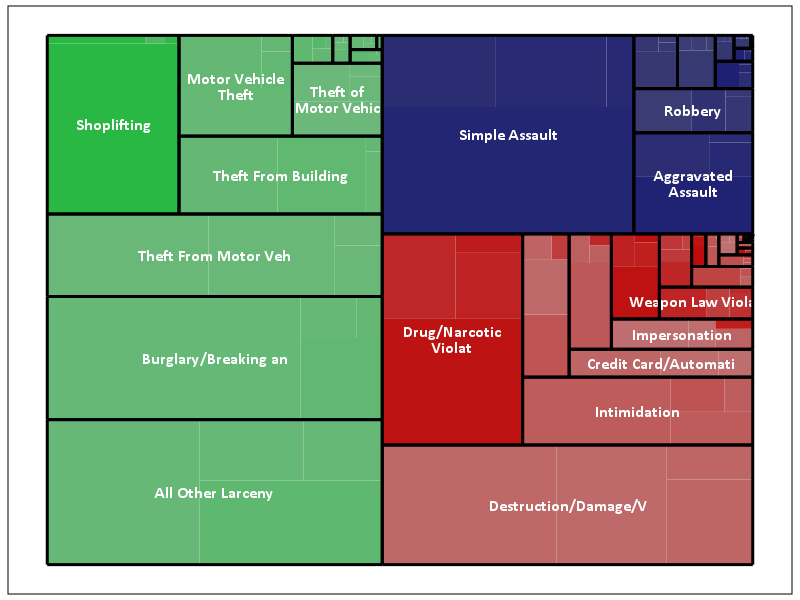This ends up being pretty boring though, there does not appear to be much variation within the location types for arrest rates. For here with widely varying category sizes I would likely want to do a model based approach and shrink the extreme proportions in the smaller categories, but that is a challenge for another blog post! (Also the sizes of the categories naturally de-emphasizes the small areas.)

One of the other things I was experimenting with was the use of svg gradients via the chart template, (see Squarified Treemaps (Bruls et al., 2000) for a motivating example) but I was unable to figure out the chart template xml needed to have the polygons drawn with gradients. (I had saved a few templates from V20 that had example gradients in them, and I’ve gotten them to work for bar graphs.) Also I attempted to export this with tooltips, but the tool tips were derived variables from the polygons, so I’m not quite sure how to cajole SPSS to give the tool tips I want.

This is not the best use of treemaps though, and I will have to write a post showing how small multiples of bar graphs can be just as effective as these examples. Shneiderman intended these to be an interactive application in which you could see the forest and then drill down into smaller subsets for exploration. Comparing areas across categories in this example, e.g. comparing the proportion of crimes occurring at home in assaults versus robberies, is very difficult to accomplish in the treemap. I would say that they are slightly more aesthetically pleasing than the wooden Charlie Brown xmas tree I built for my tiny apartment though.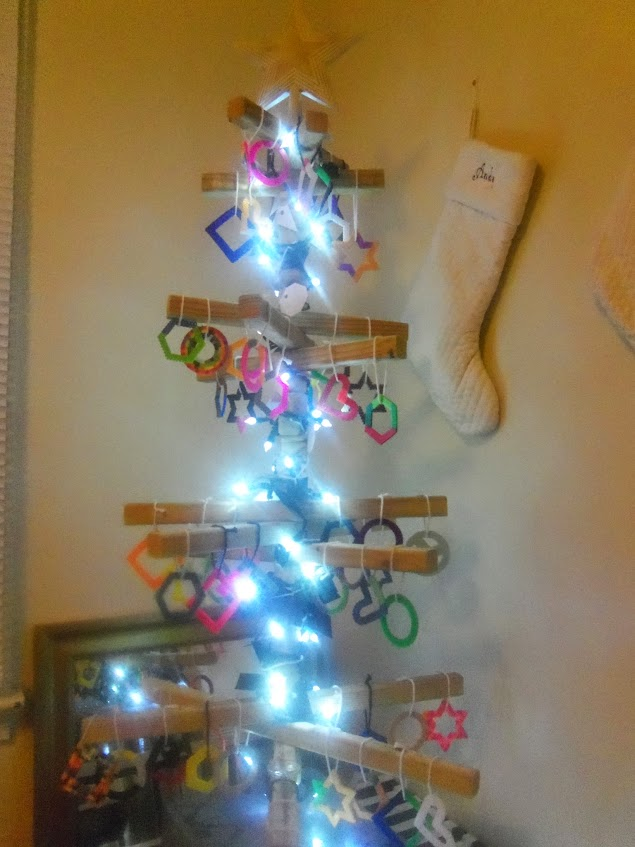Happy Holidays!